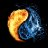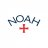# Trend Directional Force Index (TDFI) - Tradingview

C

#### ccrkk

##### New member
VIP
Hello,

I found this trend trading indicator on Tradingview and was wondering if someone could possibly convert it into a TOS study. I scrolled though charts on Tradingview with the indicator and it really did a nice job of keeping you out of trades while in a sideways market with the ".05" high/low filter. Would like to test it out on TOS to analyze it further and conduct more backtest on it. Any help would be greatly appreciated.

Below is the code from Tradingview: (All credit goes to "causecelebre" from Tradingview for this indicator)

Code:
``````//@version=4
study("Trend Direction Force Index v2 - TDFI [wm]", shorttitle = "TDFI v2 [wm]", overlay = false)

lookback = input(13, title = "Lookback")
mmaLength = input(13, title = "MMA Length")
mmaMode = input(title = "MMA Mode", defval="ema", options=["ema", "wma", "swma", "vwma", "hull", "tema"])
smmaLength = input(13)
smmaMode = input(title = "SMMA Mode", defval="ema", options=["ema", "wma", "swma", "vwma", "hull", "tema"])
nLength = input(3, title = "N Length")
filterHigh = input(0.05, title = "Filter High")
filterLow = input(-0.05, title = "Filter Low")
price = input(close, "Period")

tema(src, len) =>
ema1 = ema(src, len)
ema2 = ema(ema1, len)
ema3 = ema(ema2, len)
(3 * ema1) - (3 * ema2) + ema3

ma(mode, src, len) =>
mode == "ema" ? ema(src, len) :
mode == "wma" ? wma(src, len) :
mode == "swma" ? swma(src) :
mode == "vwma" ? vwma(src, len) :
mode == "hull" ? wma((2 * wma(src, len / 2)) - wma(src, len), round(sqrt(len))) :
mode == "tema" ? tema(src, len) :
sma(src, len)

tdfi() =>
mma = ma(mmaMode, price * 1000, mmaLength)
smma = ma(smmaMode, mma, smmaLength)
impetmma = mma - mma
impetsmma = smma - smma
divma = abs(mma - smma)
averimpet = (impetmma + impetsmma) / 2
tdf = pow(divma, 1) * pow(averimpet, nLength)
tdf / highest(abs(tdf), lookback * nLength)

signal = tdfi()
c = signal > filterHigh ? color.green : signal < filterLow ? color.red : color.gray

plot(signal, linewidth = 2, color = c)
hline(filterHigh, color = color.black)
hline(filterLow, color = color.black)``````

Z

#### Zuben

##### New member
Just saw your request and and it looks interesting, so I did a quick translate to thinkscript. Should have all the same features except TEMA, which you can switch to by adding/removing comment markers (just to keep it simple, see below). Let me know what you think.Code:
``````# thinkscript version of Tradingview's Trend Direction Force Index (TDFI)
# by Zuben

declare lower;
input lookback = 13;
input mmaLength = 13;
input smmaLength = 13;
input mmaMode = AverageType.EXPONENTIAL;
input smmaMode = AverageType.EXPONENTIAL;
input nLength = 3;
input filterHigh = 0.05;
input filterLow = -0.05;
input price = close;

def mma = MovingAverage(mmaMode, price*1000, mmaLength);
def smma = MovingAverage(smmaMode, mma, smmaLength);
#for TEMA: comment out the two above lines and uncomment the following
#def mma = TEMA(price*1000, mmaLength);
#def smma = TEMA(mma, smmaLength);
def impetmma = mma - mma;
def impetsmma = smma - smma;
def divma = AbsValue(mma - smma);
def averimpet = (impetmma + impetsmma) / 2;
def tdf = Power(divma, 1) * Power(averimpet, nLength);
def tdfi = tdf / Highest(AbsValue(tdf), lookback * nLength);

plot signal = tdfi;
plot fh = filterHigh;
plot fl = filterLow;
plot zl = 0;

signal.SetDefaultColor(GetColor(4));
fh.SetDefaultColor(GetColor(2));
fl.SetDefaultColor(GetColor(2));
zl.SetDefaultColor(GetColor(7));

signal.DefineColor("Positive", Color.UPTICK);
signal.DefineColor("Negative", Color.DOWNTICK);
signal.AssignValueColor(if signal > fh then signal.color("Positive") else if signal < fl then signal.color("Negative") else Color.ORANGE);``````

•T

##### Active member
2019 Donor
@Zuben Is it possible to add two sets of inputs? How owuld i go about that thanks.

input filterHigh = 0.05;
input filterLow = -0.05;

input filterHigh = 1.00;
input filterLow = -1.00;#### BenTen

Staff
VIP

input filterHigh2 = 1;
input filterLow2 = -1;
plot FH2 = filterHigh2;
plot FL2 = filterLow2;

•T

##### Active member
2019 Donor

input filterHigh2 = 1;
input filterLow2 = -1;
plot FH2 = filterHigh2;
plot FL2 = filterLow2;
thank you!

Z

#### Zuben

##### New member
@Trading51, This indicator is range-bound between 1 and -1, so setting those as a secondary high/low filter would just put a boundary at the top and bottom. From what I understand, the very low filters are to keep you ought of sideways markets. When it's above/below those filters, it indicates movement/momentum out of the sideways move.

But a secondary filter may have good use at a .5/-.5 range, which would give you a better idea of the strength of the move.

•T

##### Active member
2019 Donor
@Trading51, This indicator is range-bound between 1 and -1, so setting those as a secondary high/low filter would just put a boundary at the top and bottom. From what I understand, the very low filters are to keep you ought of sideways markets. When it's above/below those filters, it indicates movement/momentum out of the sideways move.

But a secondary filter may have good use at a .5/-.5 range, which would give you a better idea of the strength of the move.
Thanks4 & 20 Period Historical Volatility - Reversals and Trend Exhaustion Indicators 0Super Trend + 9 ema + VWAP Questions 3